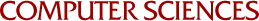## Interactive Graphical Spline Approximation to Boundary Value Problems

J.B. Rosen, Paul LaFata
1971

Earlier work on interactive graphical approximation of data using linear programming has now been extended to ordinary differential equation multipoint boundary value problems. The approximation is obtained using a suitable spline basis where the degree and uniform knot size is specified by the user. The coefficients of the spline basis are determined so as to minimize the maximum error in the differential equation over a specified discrete grid.